## 6 Functions on the receive (RX) side

06.623GPPComfort noise aspects for Enhanced Full Rate (EFR) speech traffic channelsTS

The situations in which comfort noise shall be generated on the receive side are defined in GSM 06.81 . Generally speaking, the comfort noise generation is started or updated whenever a valid SID frame is received.

## 6.1 Averaging and decoding of the LP and fixed codebook gain parameters

When speech frames are received by the decoder the LP and the fixed codebook gain parameters of the last seven speech frames shall be kept in memory. The decoder counts the number of frames elapsed since the last SID frame was updated and passed to the RSS by the encoder. Based on this count, the decoder determines whether or not there is a hangover period at the end of the speech burst (if at least 31 frames have elapsed since the last SID update when the first SID frame after a speech burst arrives, the hangover period has existed at the end of the speech burst).

As soon as a SID frame is received, and the hangover period is detected at the end of the speech burst, the stored LP and fixed codebook gain parameters shall be averaged to obtain the reference LSF parameter vectorand the reference fixed gain codebook value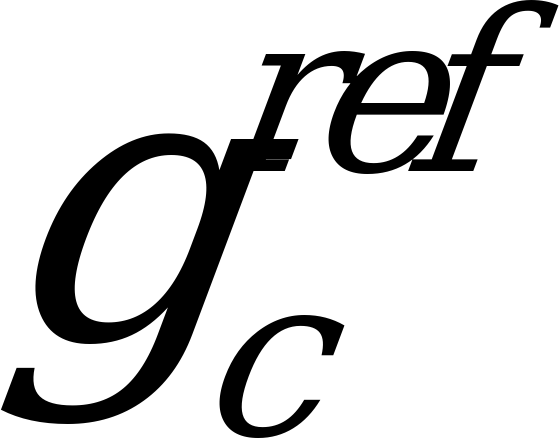. The reference LSF parameter vector and the reference fixed codebook gain value shall be frozen and used for the actual comfort noise insertion period.

The averaging procedure for obtaining the reference parameters is as follows:

– when a speech frame is received, the LSF and fixed codebook gain parameters are decoded and stored in memory;

– when the first SID frame is received, and the hangover period is detected at the end of the speech burst, the stored LSF and fixed codebook gain parameters are averaged in the same way as in the speech encoder as follows (see also clause 5.1):

(8)

where:

is the mth quantized LSF parameter vector of one of the frames of the hangover period
(n = 1,…, 7);

n is the hangover period frame index (n = 1, 2,…, 7);

m is the LSF parameter index within a frame (1 or 2);

k is the frame index.

and

(9)

where:

is the quantized fixed codebook gain parameter value in subframe j of one of the frames of the hangover period (n = 1,…, 7);

n is the hangover period frame index (n = 1,…, 7);

k is the frame index;

j is the subframe index (j = 1,…, 4).

Once the reference LSF parameter vector has been computed, the averaged LSF parameter vector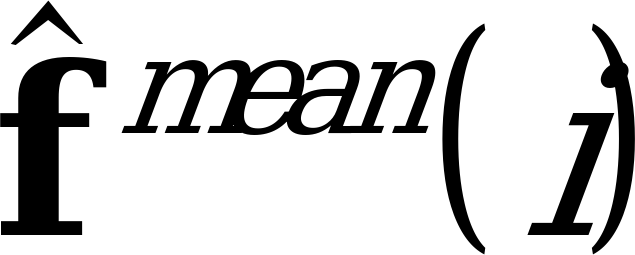of the frame i (encoded into the SID frame) can be reproduced at the decoder each time a SID update frame is received, according to the equation: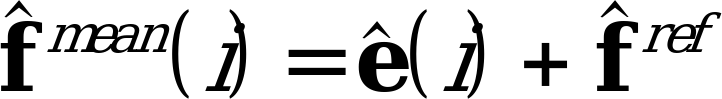(10)

where: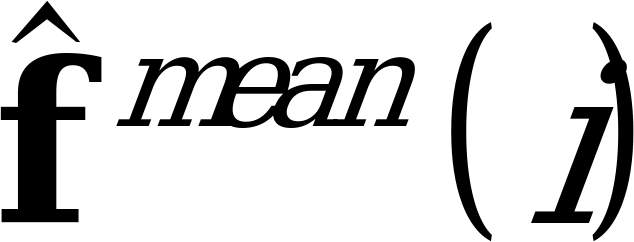is the quantized, averaged LSF parameter vector at the current frame i to be used for comfort noise generation;is the reference LSF parameter vector;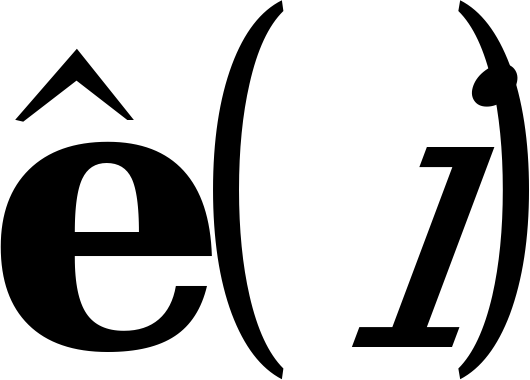is the received quantized LSF parameter prediction residual at the current frame i;

i is the frame index.

The averaged fixed codebook gain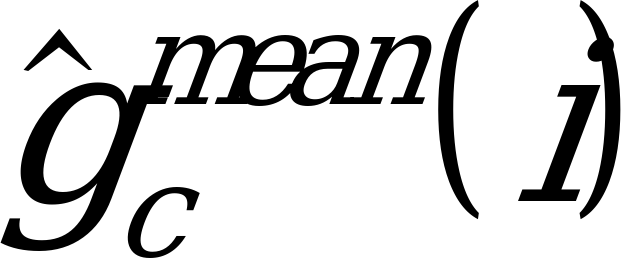of the frame i (encoded into the SID frame) can be similarly reproduced at the decoder each time a SID update frame is received, according to the following equation: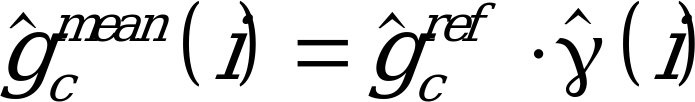(11)

where: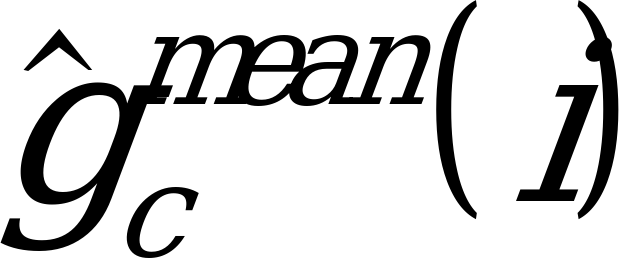is the averaged fixed codebook gain value in the current frame i to be used for comfort noise generation;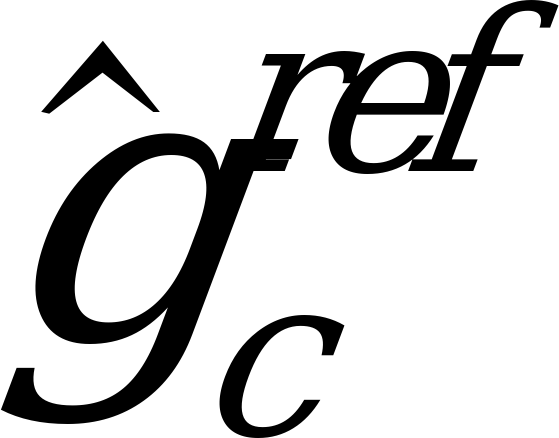is the reference fixed codebook gain;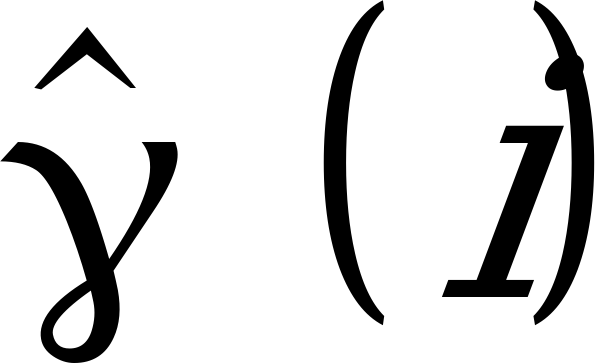is the received quantized fixed codebook gain correction factor in the current frame i;

i is the frame index.

## 6.2 Comfort noise generation and updating

The comfort noise generation procedure uses the GSM Enhanced Full Rate speech decoder algorithm defined in GSM 06.60 .

When comfort noise is to be generated, the various encoded parameters are set as follows:

In each subframe, the pulse positions and signs of the fixed codebook excitation are locally generated using uniformly distributed pseudo random numbers. The excitation pulses take values of +1 and ‑1 when comfort noise is generated. The fixed codebook comfort noise excitation generation algorithm works as follows:

for (i = 0; i < 40; i++) code[i] = 0;

for (i = 0; i < 10; i++) {

j = random(4);

idx = j * 10 + I;

if (random(2) == 1) code[idx] = 1;

else code[idx] = ‑1;

}

where:

code[0..39] fixed codebook excitation buffer;

random(4) generates a random integer value, uniformly distributed between 0 and 3;

random(2) generates a random integer value, uniformly distributed between 0 and 1.

The fixed codebook gain values are those received in the SID frame.

The adaptive codebook gain values in each subframe are set to 0.

The pitch delay values in each subframe are set to 40.

The LP filter parameters used are those received in the SID frame.

The predictor memories of the ordinary LP parameter and fixed codebook gain quantization algorithms are reset when SP flag = "0", so that the quantizers start from their initial states when the speech activity begins again.

With these parameters, the speech decoder now performs the standard operations described in GSM 06.60  and synthesizes comfort noise.

Updating of the comfort noise parameters (fixed codebook gain and LP filter parameters) occurs each time a valid SID frame is received, as described in GSM 06.81 .

When updating the comfort noise, the parameters above should preferably be interpolated over the SID update period to obtain smooth transitions.